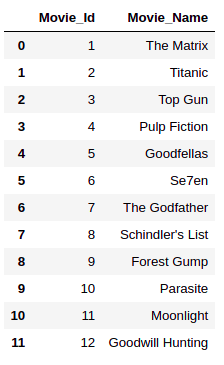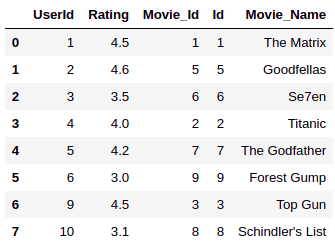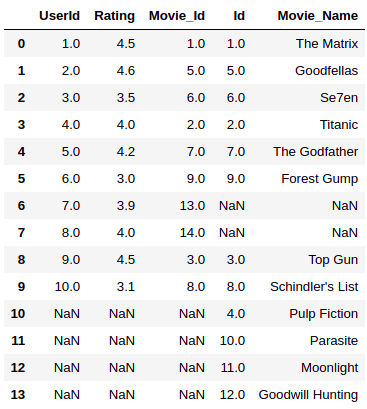Merging dataframes in Pandas is an important data manipulation technique. In this tutorial, we’re going to show you several ways to merge dataframes using the `merge()` function in Python Pandas.

In an earlier tutorial, we demonstrated how to use Pandas concat and how to use Pandas join functions for concatenating and merging Pandas dataframes. The `merge()` function is another way you can combine dataframes.

The main difference between the `merge()` and `join()` functions is that `join` is primarily used for combining dataframes using indices whereas the `merge()` function can be used for combining dataframes using common values in columns and indices. Finally, the `concat()` function is simply used for appending dataframes horizontally or vertically, based on the axis attribute.

## How to use the Pandas Merge Function

Let’s see a simple example of the `merge()` function in Pandas.

The following script creates a dummy pandas dataframe named user_df with three columns:

``````import pandas as pd

user_id = [1, 2, 3, 4, 5, 6, 7, 8, 9, 10]
user_ratings = [4.5, 4.6, 3.5, 4.0, 4.2, 3.0, 3.9, 4.0, 4.5, 3.1]
movie_id = [1,5,6,2,7,9,13,14,3,8]

user_df = pd.DataFrame(
list(zip(user_id, user_ratings, movie_id)),
columns =['UserId', 'Rating', 'Movie_Id']
)
user_df``````

Output:The script below creates another dummy dataframe movie_df with two columns:

``````movie_id = [1,2,3,4,5,6,7,8,9,10,11,12]
movie_name =["The Matrix", "Titanic", "Top Gun","Pulp Fiction",
"Goodfellas","Se7en", "The Godfather", "Schindler's List",
"Forest Gump", "Parasite", "Moonlight", "Goodwill Hunting"]

movie_df = pd.DataFrame(
list(zip(movie_id, movie_name)),
columns =['Movie_Id', 'Movie_Name']
)
movie_df``````

Output:To merge the user_df and movie_df dataframes you need to call the `merge()` function on one of the dataframes, and pass it the second dataframe, as shown in the script below. If you do not pass any other parameter to the `merge()` function, by default the dataframes will be merged using matching column names.

Since the “Movie_Id” column is the matching column in our user_df and movie_df dataframes, all the rows from both the dataframes will be returned where a match is found. This type of merge is called merge by inner join and is similar to the inner join operation in SQL.

``````merged_df = user_df.merge(movie_df)

merged_df``````

Output:Another way to merge two dataframes is by calling the `pd.merge()` function and passing it the two dataframes you want to merge. Take a look at this example:

``````import pandas as pd

merged_df = pd.merge(user_df, movie_df)

merged_df``````

Output:I put together a Python Developer Kit with over 100 pre-built Python scripts covering data structures, Pandas, NumPy, Seaborn, machine learning, file processing, web scraping and a whole lot more - and I want you to have it for free. Enter your email address below and I'll send a copy your way.

Yes, I'll take a free Python Developer Kit

## Merging DataFrames by Column Names

What do you do if your dataframes don’t have columns with matching names? In this scenario, you’ll have to specify the column names you want to use to merge your dataframes.

Let’s modify our movie_df dataframe and rename the “Movie_Id” column to “Id”

``````movie_id = [1,2,3,4,5,6,7,8,9,10,11,12]
movie_name =["The Matrix", "Titanic", "Top Gun","Pulp Fiction",
"Goodfellas","Se7en", "The Godfather", "Schindler's List",
"Forest Gump", "Parasite", "Moonlight", "Goodwill Hunting"]

movie_df = pd.DataFrame(
list(zip(movie_id, movie_name)),
columns =['Id', 'Movie_Name']
)
movie_df``````

Output:Now our user_df and movie_df dataframes have no columns with matching names. To merge our dataframes now, we’ll have to take a different approach.

We’re going to show you four different types of JOIN operations you can perform using the `merge()` functions in Pandas. These JOIN operations are similar to SQL JOINs.

### Merging with Inner Join

You’ve already seen an example of an inner join merge operation in the last section. This is the default when you don’t pass any parameters to the Pandas `merge()` function except the names of the dataframes you want to merge.

If the matching columns in the two dataframes to be merged have different names, you need to pass values for the `left_on` and `right_on` parameters of the `merge()` function. The columns name to be matched from the left dataframe (“Movie_Id” column from the user_df in the following script) is passed to `left_on` parameter. The column name from the right dataframe (“Id” column from the movie_df in the following script) is passed to the `right_on` parameter of the `merge()` function.

``````merged_df = user_df.merge(movie_df, left_on = "Movie_Id", right_on = "Id")

merged_df``````

Output:If two dataframes have the same column name, you can also pass the matching name to the `on` parameter of the `merge()` function.

### Merging with Left Join

The merge with left join in Pandas is similar to the SQL left join operation. When merging with a left join, all the rows from the left table are returned. For the rows in the right table where there are no matching values in the matching column, NULL values are returned.

To perform the left join operation, you need to pass “left” as the value for the `how` parameter of the `merge()` function, just like this:

``````merged_df = user_df.merge(movie_df, left_on = "Movie_Id", right_on = "Id", how = "left" )

merged_df``````

Output:The above output shows all the rows from the user_df dataframe. In the rows for the “Id” column of the movie_df dataframe, NULL (NaN) values are added where no match is found between it and the “Movie_Id” column of the user_df dataframe.

### Merging with Right joins

The merge with right join in Pandas is the same as the SQL right join operation. Merging with a right join returns all the rows from the right table. NULL values are returned from the left table where there are no matching values in the column you’re trying to join.

To perform the left join operation, you need to pass “right” as the value for the `how` parameter of the `merge()` function. Here’s a basic right join example to get you started:

``````merged_df = user_df.merge(movie_df, left_on = "Movie_Id", right_on = "Id", how = "right")

merged_df``````

Output:### Merging with Outer joins

Merging Pandas DataFrames with an outer join returns all the rows from both the tables involved in the merge operations. NULL values are returned for the matching columns of the two dataframes in cases where no matches are found.

``````merged_df = user_df.merge(movie_df, left_on = "Movie_Id", right_on = "Id", how = "outer" )

merged_df``````

Output:### Merging DataFrames by Indexes

In addition to merging tables by column names, you can also merge tables by index values. To do this, you need to pass True as values for the `left_index` and `right_index` attributes of the merge function. Take a look:

``````merged_df = user_df.merge(movie_df, left_index = True, right_index = True)

merged_df``````

Output:Since the user_df table only has indices from 0 to 9, while the movie_df table contains indexes from 0-12, the rows for the matching indexes 0-9 are returned from both tables. Notice how the “Movie_Id” and “Id” columns no longer match since we merged based on matching index values (row names) instead of column names.